# Volumes of Cylinders

In this worksheet, students calculate the volume of cylinders given their diameter and height.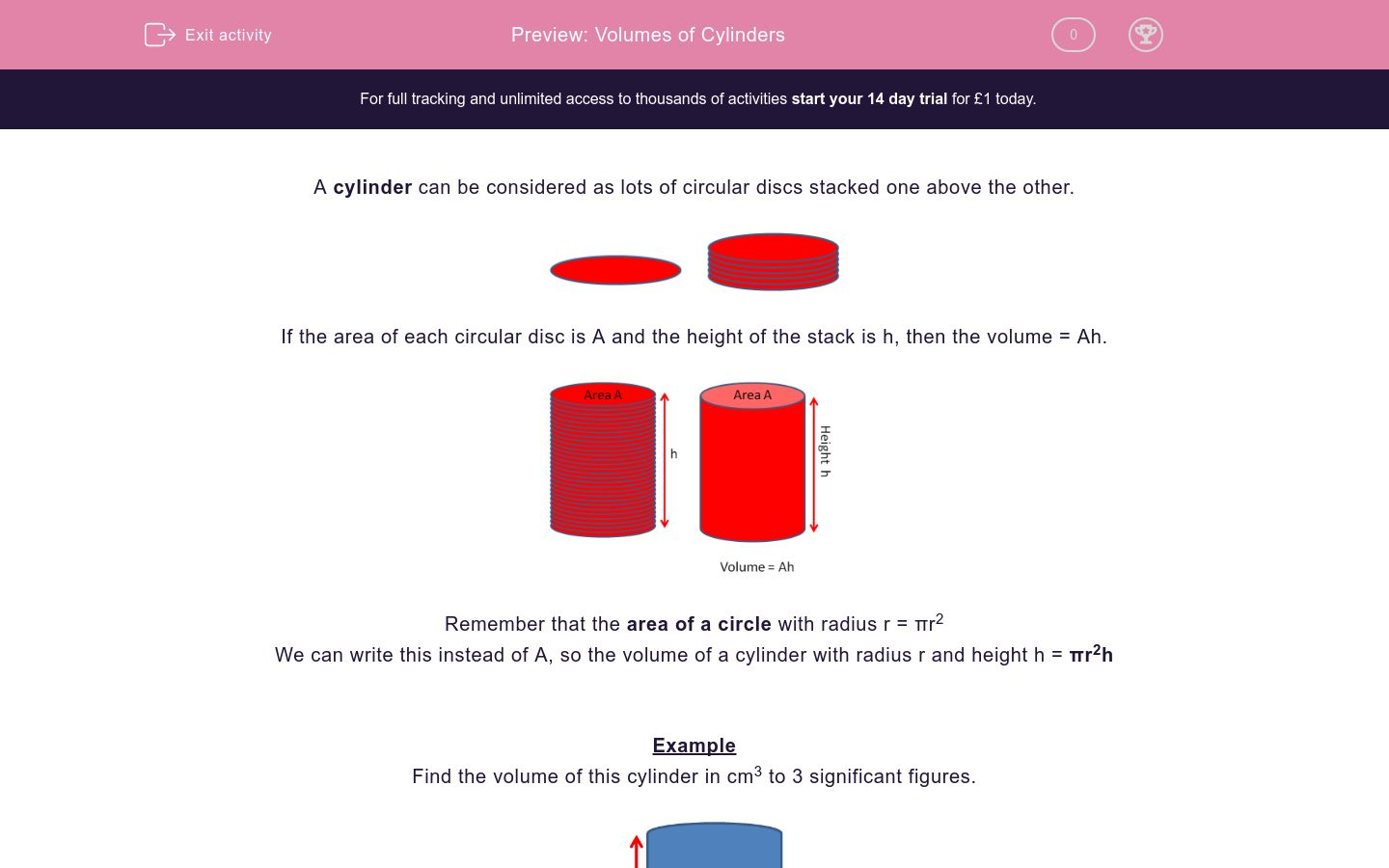Key stage:  KS 2

Curriculum topic:   Maths and Numerical Reasoning

Curriculum subtopic:   Volume and Capacity

Difficulty level:### QUESTION 1 of 10

A cylinder can be considered as lots of circular discs stacked one above the other.If the area of each circular disc is A and the height of the stack is h, then the volume = Ah.Remember that the area of a circle with radius r = πr2

We can write this instead of A, so the volume of a cylinder with radius r and height h = πr2h

Example

Find the volume of this cylinder in cm3 to 3 significant figures.Diameter = 6 cm

Radius = 6 ÷ 2 = 3 cm

Height = 12 cm

Volume = πr2h = π × 32 × 12 = 339.29 ≈ 339 cm3 (3 s.f.)

Find the volume of this cylinder in cm3 to 3 significant figures.

(just write the number)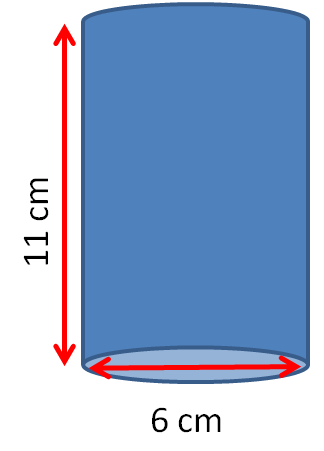Find the volume of this cylinder in cm3 to 3 significant figures.

(just write the number)Find the volume of this cylinder in cm3 to 3 significant figures.

(just write the number)Find the volume of this cylinder in cm3 to 3 significant figures.

(just write the number)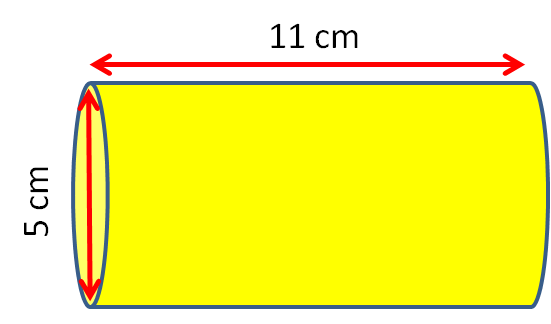Find the volume of this cylinder in cm3 to 3 significant figures.

(just write the number)Find the volume of this cylinder in cm3 to 3 significant figures.

(just write the number)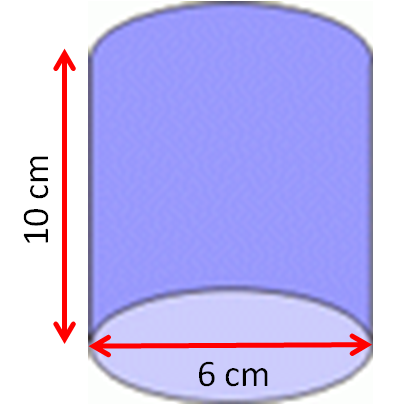Find the volume of this cylinder in cm3 to 3 significant figures.

(just write the number)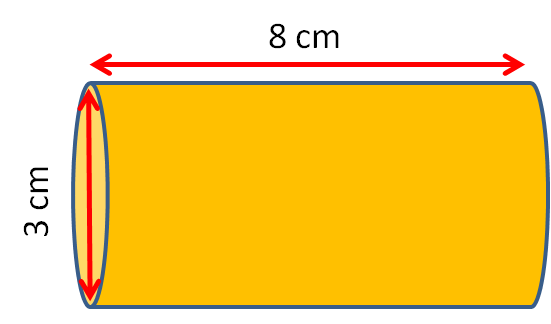Find the volume of this cylinder in cm3 to 3 significant figures.

(just write the number)Find the volume of this cylinder in cm3 to 3 significant figures.

(just write the number)Find the volume of this cylinder in cm3 to 3 significant figures.

(just write the number)• Question 1

Find the volume of this cylinder in cm3 to 3 significant figures.

(just write the number)311
EDDIE SAYS
The diamater is 6, so we need to half it to find the radius. π × 3² × 11
• Question 2

Find the volume of this cylinder in cm3 to 3 significant figures.

(just write the number)503
EDDIE SAYS
The diamater is 8, so we need to half it to find the radius π × 4² × 10
• Question 3

Find the volume of this cylinder in cm3 to 3 significant figures.

(just write the number)475
EDDIE SAYS
The diamater is 11, so we need to half it to find the radius π × 5.5² × 5
• Question 4

Find the volume of this cylinder in cm3 to 3 significant figures.

(just write the number)216
EDDIE SAYS
The diamater is 5, so we need to half it to find the radius π × 2.5² × 11
• Question 5

Find the volume of this cylinder in cm3 to 3 significant figures.

(just write the number)1410
EDDIE SAYS
The diamater is 15, so we need to half it to find the radius π × 7.5² × 8
• Question 6

Find the volume of this cylinder in cm3 to 3 significant figures.

(just write the number)283
EDDIE SAYS
The diamater is 6, so we need to half it to find the radius π × 3² × 10
• Question 7

Find the volume of this cylinder in cm3 to 3 significant figures.

(just write the number)56.5
EDDIE SAYS
The diamater is 3, so we need to half it to find the radius π × 1.5² × 8
• Question 8

Find the volume of this cylinder in cm3 to 3 significant figures.

(just write the number)113
EDDIE SAYS
The diamater is 4, so we need to half it to find the radius π × 2² × 9
• Question 9

Find the volume of this cylinder in cm3 to 3 significant figures.

(just write the number)1820
EDDIE SAYS
The diamater is 17, so we need to half it to find the radius π × 8.5² × 8
• Question 10

Find the volume of this cylinder in cm3 to 3 significant figures.

(just write the number)614
EDDIE SAYS
The diamater is 12.5, so we need to half it to find the radius π × 6.25² × 5
---- OR ----

Sign up for a £1 trial so you can track and measure your child's progress on this activity.

### What is EdPlace?

We're your National Curriculum aligned online education content provider helping each child succeed in English, maths and science from year 1 to GCSE. With an EdPlace account you’ll be able to track and measure progress, helping each child achieve their best. We build confidence and attainment by personalising each child’s learning at a level that suits them.

Get started